## Risk neutral probability of default

The risk neutral probability of default is a very important concept that is used mainly to price derivatives and bonds. The risk neutral probability is defined as the default rate implied by the current market price. In general, the estimated risk neutral default probability will correlate positively with the recovery rate.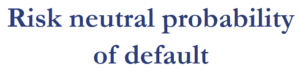In what follows, we discuss a simple example that explains how to calculate the risk neutral probability. We use an Excel spreadsheet to implement the approach.

## Risk neutral probability of default example

Let’s consider, for simplicity, a zero-coupon bond. It has a par value of $100 and is currently trading at$95. The benchmark 1-year rate is 3% an the recovery rate is approximately 60%. Now, what are the possible outcomes? At the end of the year, either the bond does not default, in which case we receive $100 or it does default. If it defaults, we will only recover 60% of a$100, or \$60.

Let’s denote the probability of default using p. In this case, the expected year-end cash flow equals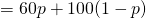We need to discount this cash flow back to today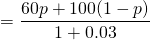Finally, let’s set this value equal to the market price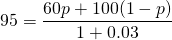Solving for p, we find that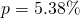This is the risk-neutral probability. It is important that we assumed the recovery rate will be 60%. Different recovery rates will result in different probabilities of default. The same methodology can also be used to calculate the implied recovery rate. In that case, we assume a certain risk-neutral probability of default p and solve for the recovery rate. Also, had we assumed a higher recovery rate, then the probability of default would have been higher (for the same market price).

## Summary

We discussed how to calculate the risk neutral default probability using a security’s market price. We also discussed that it is possible to calculate the implied recovery rate. These method are often used in the context of credit modelling.